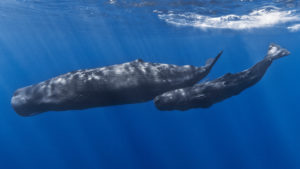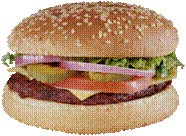## Units of Weight

### LEARNING outcomes

• Define units of length and convert from one to another.
• Perform arithmetic calculations on units of length.
• Solve application problems involving units of length.
• Define units of weight and convert from one to another.
• Perform arithmetic calculations on units of weight.
• Solve application problems involving units of weight.
• Describe the general relationship between the U.S. customary units and metric units of length, weight/mass, and volume.
• Define the metric prefixes and use them to perform basic conversions among metric units.
• Solve application problems involving metric units of length, mass, and volume.
• State the freezing and boiling points of water on the Celsius and Fahrenheit temperature scales.
• Convert from one temperature scale to the other, using conversion formulas.

When you mention how heavy or light an object is, you are referring to its weight. In the U.S. customary system of measurement, weight is measured in ounces, pounds, and tons. These measurements actually refer to how much the gravitational force of the Earth pulls on the object. Like other units of measurement, you can convert between these units and you sometimes need to do this to solve problems.

The grocery store sells a 36 ounce canister of ground coffee for $14, and sells bulk coffee for$9 per pound. Which is the better deal? To answer this question, you need to understand the relationship between ounces and pounds.

You often use the word weight to describe how heavy or light an object or person is. Weight is measured in the U.S. customary system using three units: ounces, pounds, and tons. An ounce is the smallest unit for measuring weight, a pound is a larger unit, and a ton is the largest unit.

 Whales are some of the largest animals in the world. Some species can reach weights of up to 200 tons–thats equal to 400,000 pounds.Meat is a product that is typically sold by the pound. One pound of ground beef makes about four hamburger patties.Ounces are used to measure lighter objects. A stack of 11 pennies is equal to about one ounce.You can use any of the customary measurement units to describe the weight of something, but it makes more sense to use certain units for certain purposes. For example, it makes more sense to describe the weight of a human being in pounds rather than tons. It makes more sense to describe the weight of a car in tons rather than ounces.

1 pound = 16 ounces

$\frac{16\text{ ounces}}{1\text{ pound}}$

## Converting Between Units of Weight

Four ounces is a typical serving size of meat. Since meat is sold by the pound, you might want to convert the weight of a package of meat from pounds to ounces in order to determine how many servings are contained in a package of meat.

The weight capacity of a truck is often provided in tons. You might need to convert pounds into tons if you are trying to determine whether a truck can safely transport a big shipment of heavy materials.

The table below shows the unit conversions and conversion factors that are used to make conversions between customary units of weight.

Unit Equivalents Conversion Factors (heavier to lighter units of measurement) Conversion Factors(lighter to heavier units of measurement)

1 pound = 16 ounces

$\frac{16\text{ ounces}}{1\text{ pound}}$

$\displaystyle \frac{\text{1 pound}}{\text{16 ounces}}$

1 ton = 2000 pounds

$\frac{2000\text{ pounds}}{1\text{ ton}}$

$\displaystyle \frac{\text{1 ton}}{\text{2000 pounds}}$

You can use the factor label method to convert one customary unit of weight to another customary unit of weight. This method uses conversion factors, which allow you to cancel units to end up with your desired unit of measurement.

Each of these conversion factors is a ratio of equal values, so each conversion factor equals 1. Multiplying a measurement by a conversion factor does not change the size of the measurement at all, since it is the same as multiplying by 1. It just changes the units that you are using to measure it in.

Two examples illustrating the factor label method are shown below.

### Exercises

How many ounces are in $\displaystyle 2\frac{1}{4}$ pounds?

### Example

How many tons is 6,500 pounds?

## Applications of Unit Conversions With Weight

There are times when you need to perform calculations on measurements that are given in different units. To solve these problems, you need to convert one of the measurements to the same unit of measurement as the other measurement.

Think about whether the unit you are converting to is smaller or larger than the unit you are converting from. This will help you be sure that you are making the right computation. You can use the factor label method to make the conversion from one unit to another.

Here is an example of a problem that requires converting between units.

### Example

A municipal trash facility allows a person to throw away a maximum of 30 pounds of trash per week. Last week, 140 people threw away the maximum allowable trash. How many tons of trash did this equal?

### Try It

Let’s revisit the coffee price problem that was posed earlier. We can use unit conversion to solve this problem.

### Example

The grocery store sells a 36 ounce canister of ground coffee for $14, and sells bulk coffee for$7 per pound. Which is the better deal?

### Try It

The average weight of a northern bluefin tuna is 1,800 pounds. The average weight of a great white shark is $\displaystyle 2\frac{1}{2}$ tons. On average, how much more does a great white shark weigh, in pounds, than a northern bluefin tuna?

## Summary

In the U.S. customary system of measurement, weight is measured in three units: ounces, pounds, and tons. A pound is equivalent to 16 ounces, and a ton is equivalent to 2,000 pounds. While an objects weight can be described using any of these units, it is typical to describe very heavy objects using tons and very light objects using an ounce. Pounds are used to describe the weight of many objects and people.

Often, in order to compare the weights of two objects or people or to solve problems involving weight, you must convert from one unit of measurement to another unit of measurement. Using conversion factors with the factor label method is an effective strategy for converting units and solving problems.